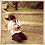222

## Latest \$type=blogging\$count=5\$author=hide\$comment=hide\$label=hide\$date=hide\$show=home

Cost of equity share capital is that part of cost of capital which is payable to equity shareholder. Every shareholder gets shares for getting return on it. So, for company point of view, it will be cost and company must earn more than cost of equity capital in order to leave unaffected the market value of its shares.

We can calculate cost of equity capital with following ways:

1. Dividend yield method or Dividend Price ratio method

According to dividend yield method or dividend price ratio method, “Cost of equity capital is minimum rate which will be equal to the present value of future dividend per share with current price of a share.

Cost of equity =

Dividend per equity share/ Market price or net proceed of per share

Ke = DPS/ MPPS or NPPS

A company issues 1000 shares of Rs. 100 each at a premium of 10%. The company has been paying 20% dividend to equity shareholders for the past five years and expects to maintain the same in the future also. Compute the cost of equity capital. Will it make any difference if the market price of equity share is Rs. 160?

Solution:

Ke= DPS/MPPS or NPPS

= 20/110 X 100

= 18.18%

If the market price of equity share is Rs. 160.

=20/160 X 100 = 12.5%

At increasing of market price, value of cost of equity capital will decrease.

2. Dividend yield plus growth in dividend method

Dividend yield plus growth in dividend method is based on the assumption that company is growing and its shares market value is also increasing. In that situation, shareholders want more than simple dividend, so company can provide some more profit according to growth. So, we will add it in previous calculated cost of equity capital.

Cost of Equity Capital = DPS/ MPPS or NPPS + Rate of growth in dividends

Ke = DPS/ MPPS or NPPS + G%

A company plans to issue 1000 new equity shares of Rs. 100 each at par. The floatation costs are expected to be 5% of the share price. The company pays a dividend of Rs. 10 per share initially and the growth in dividends is expected to be 5%. Compute the cost of new issue of equity shares.

= 10/100-5 + 5% = 15.53%

If the current market price of equity share is Rs. 150, calculate the cost of existing equity share capital.

=10/150 +5% = 11.67%

3. Earning yield method

According to this method, cost of equity capital is minimum rate which we have to earn on market price of a share. Its formula is

Ke = Earning per share / Net proceed or Market price per share

Ke = EPS/ MPPS or NPPS

A firm is considering an expenditure of Rs. 60 lakhs for expanding its operations. The relevant information is as follows:

Number of existing equity shares = 10 Lakhs

Market value of existing share= Rs. 60

Net Earning = Rs. 90 Lakh

Compute the cost of existing equity share capital and of new equity capital assuming that new shares will be issued at a price of Rs. 52 per share and the costs of new issue will be Rs. 2 per share.

Earning per share = earning / total number of shares = 90/10

= Rs. 9

Ke = 9/60 X 100

Ke = 15%

Cost of new equity capital

Ke = 9/52-2 X 100 = 18%

4. Realised yield method

One of major limitation of dividend yield method or earning yield method that both methods are based on estimation of future dividend or earning. There are large numbers of factors which are uncontrollable and uncertain. And if any financial risk will happen, we can not use it in future planning or we also can not take any decision related to estimation of return on investment. So, realised yield method is best method for calculating the cost of equity share capital.

This method is based on actual earning earned on all amount of investment. After this, we try to know, how much money is financed from equity share capital and reserve amount of past profits and after this we calculate cost of equity share capital.

Ke = Actual earning per Share / Market price per share X 100

: 1
1.when i used this method to calculated WACC, i find the actual EPS is negative so i have the value of 'Ke' negative too and that impact to value of WACC where is that negative..
how to calculate 'Ke' when the value of actual EPS is negative using this method?

In comment, you can give your feedback, reviews, ideas for improving content or ask question relating to written content.

## Unlock Financial Success!

Discover the secrets of wise finance decisions in our latest ebook.

Name